﻿ 基于主成分分析模型和BP神经网络模型在钢水“脱氧合金化”配料方案优化中的应用和研究

# 基于主成分分析模型和BP神经网络模型在钢水“脱氧合金化”配料方案优化中的应用和研究Application and Research of Principal Component Analysis Model and BP Neural Network Model in Optimization of “Deoxidation Alloying” Batching Scheme for Molten Steel

Abstract: Steel is closely related to the industrial development of a country. With the advent of Manufacturing 2025, the demand for steel is further improved. Therefore, it is very important to propose a “deoxidizing alloying” solution for molten steel, which is very important for the development of industrial manufacturing and economic society. In this paper, the relationship between the historical yield of C and Mn in molten steel and other influencing factors is considered. The historical data is preprocessed, and the principal component analysis model is established to analyze the impact of the two elements. The main factors are then constructed BP neural network to predict the yield of C and Mn elements, and then improve the prediction results by using fractional order to improve the prediction results. Finally, use the idea of dynamic programming to find out Optimal cost and ingredient planning. Finally, combined with the research results of this paper, the recommendations and strategies for optimizing the ingredients are formulated.

1. 引言

2. 问题分析

1) 在钢水的脱氧合金化过程中主要关注C、Si，Mn，P，S这五种元素，根据附加中提供的数据，计算出C、Mn两种元素的历史收得率，并分析数据，找出影响收得率的主要原因。

2) 在上文的基础上，对C、Mn两种元素的收得率做出预测，并对其做出改进和优化，提高收得率预测的准确性。

3) 根据合金收得率的预测结果及历史炼钢所记录下的数据，以实现钢水脱氧合金化成本优化计算，并提供一套配料优化方案。

3. 模型的建立

3.1. 收得率计算模型

3.1.1. 数据预处理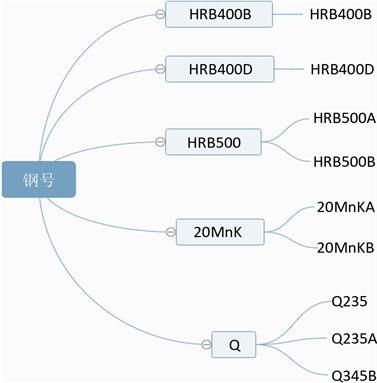Figure 1. Steel number classification map

3.1.2. C的收得率计算模型(1)

3.1.3. Mn的收得率计算模型

$\left\{\begin{array}{l}p=\frac{\left({n}_{1}-{n}_{0}\right)\ast {m}_{钢}}{M}\\ M={m}_{Mn}+{m}_{钢}×{n}_{0}\\ {m}_{Mn}={m}_{合金}×{n}_{c}\end{array}$ (2)

3.2. 主成分分析模型

3.2.1. 对原始数据进行标准化处理

$\left\{\begin{array}{l}{\stackrel{˜}{a}}_{ij}=\frac{{a}_{ij}-{\mu }_{j}}{{s}_{j}},i=1,2,\cdots ,n\\ {\mu }_{j}=\frac{1}{n}\underset{i=1}{\overset{n}{\sum }}{a}_{ij}\\ {s}_{j}=\sqrt{\frac{1}{n-1}\underset{i=1}{\overset{n}{\sum }}{\left({a}_{ij}-{\mu }_{j}\right)}^{2}}\\ j=1,2\cdots ,m\end{array}$ (3)

3.2.2. 计数相关系数矩阵R

$\left\{\begin{array}{l}{r}_{ij}=\frac{\underset{i=1}{\overset{n}{\sum }}{\stackrel{˜}{a}}_{ki}\cdot {\stackrel{˜}{a}}_{kj}}{n-1},\text{\hspace{0.17em}}\text{\hspace{0.17em}}i,j=1,2,\cdots ,m\\ {r}_{ii}=1\\ {r}_{ij}={r}_{ji}\end{array}$ (4)

3.2.3. 计算特征值和特征向量

$\begin{array}{l}{y}_{1}={u}_{11}{\stackrel{˜}{x}}_{1}+{u}_{21}{\stackrel{˜}{x}}_{2}+\cdots +{u}_{m1}{\stackrel{˜}{x}}_{m},\\ {y}_{2}={u}_{12}{\stackrel{˜}{x}}_{1}+{u}_{22}{\stackrel{˜}{x}}_{2}+\cdots +{u}_{m2}{\stackrel{˜}{x}}_{m},\\ \text{\hspace{0.17em}}\text{\hspace{0.17em}}\text{\hspace{0.17em}}\text{\hspace{0.17em}}\text{\hspace{0.17em}}\text{\hspace{0.17em}}\text{\hspace{0.17em}}\text{\hspace{0.17em}}\text{\hspace{0.17em}}\text{\hspace{0.17em}}\text{\hspace{0.17em}}\text{\hspace{0.17em}}\text{\hspace{0.17em}}\text{\hspace{0.17em}}\text{\hspace{0.17em}}\text{\hspace{0.17em}}\text{\hspace{0.17em}}\text{\hspace{0.17em}}\text{\hspace{0.17em}}⋮\\ {y}_{m}={u}_{1m}{\stackrel{˜}{x}}_{1}+{u}_{2m}{\stackrel{˜}{x}}_{2}+\cdots +{u}_{mm}{\stackrel{˜}{x}}_{m},\end{array}$ (5)

3.2.4. 选择 $p\left(p\le m\right)$ 个主成分，计算综合评价值

计算特征值 ${\lambda }_{j}\left(j=1,2,\cdots ,m\right)$ 的信息贡献率和累计贡献率。称

${b}_{j}=\frac{{\lambda }_{j}}{\underset{k=1}{\overset{m}{\sum }}{\lambda }_{k}},j=1,2,\cdots ,m$ (6)

${\alpha }_{p}=\frac{\underset{k=1}{\overset{p}{\sum }}{\lambda }_{k}}{\underset{k=1}{\overset{m}{\sum }}{\lambda }_{k}}$ (7)

计算综合得分：

$z=\underset{j=1}{\overset{p}{\sum }}{b}_{j}{y}_{j}$ (8)

${b}_{j}$ 为第j个主成分的信息贡献率，根据综合得分值就可进行综合评价。

3.3. BP神经网络模型的建立

BP神经网络如图2所示，其中 ${N}_{1}$ 为输入层， ${N}_{2}$ 为中间层， ${N}_{3}$ 为输出层。本文将影响合金的相关影响因子作为输入层(如连铸正样Ceq_val、连铸正样Cr、连铸正样Ni_val连铸正样Cu_val、连铸正样V_val、连铸正样Alt_val等)，最终输出预测结果。在神经网络中，神经元接收到来自其他神经元的输入信号，这些信号乘以权重累加到神经元接收的总输入值上，随后与当前神经元的阈值进行比较，然后通过激活函数处理，即可产生神经元的输出。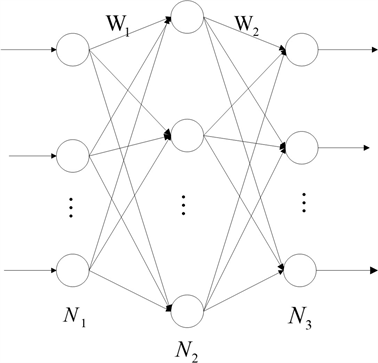Figure 2. Neural network

$sigmoid\left(x\right)=\frac{1}{1+{\text{e}}^{-x}}$ (9)

$y=\sigma \left(\left[\begin{array}{ccc}{w}_{11}& \cdots & {w}_{1n}\\ ⋮& \ddots & ⋮\\ {w}_{m1}& \cdots & {w}_{mn}\end{array}\right]\left[\begin{array}{c}\epsilon \left(1\right)\\ ⋮\\ \epsilon \left(n\right)\end{array}\right]+\left[\begin{array}{c}{\theta }_{1}\\ ⋮\\ {\theta }_{n}\end{array}\right]\right)$

$E=\frac{1}{m}\underset{i=1}{\overset{m}{\sum }}{\left(\stackrel{^}{\epsilon }\left(i\right)-\epsilon \left(i\right)\right)}^{2}$ (10)

$\left\{\begin{array}{l}O\left(i\right)=f\left(\underset{j=1}{\overset{n}{\sum }}{\omega }_{1ij}\epsilon \left(j\right)-{\theta }_{1i}\right),i=1,2,\cdots ,l\\ \stackrel{^}{\epsilon }\left(i\right)=f\left(\underset{j=1}{\overset{l}{\sum }}{\omega }_{2ij}O\left(j\right)-{\theta }_{1j}\right),i=1,2,\cdots ,m\end{array}$ (11)

3.3.1. 分数阶灰色模型

${x}^{\left(r\right)}\left(k\right)=\underset{i=1}{\overset{k}{\sum }}{C}_{k-i+r-1}^{k-i}{x}^{\left(0\right)}\left(i\right)$ (12)

${x}^{\left(r\right)}\left(k+1\right)={\beta }_{1}{x}^{\left(r\right)}\left(k\right)+{\beta }_{2}$ (13)

$\left[\begin{array}{l}{\beta }_{2}\\ {\beta }_{1}\end{array}\right]={\left({B}^{\text{T}}B\right)}^{-1}{B}^{\text{T}}Y$ (14)

${x}^{\left(-r\right)}\left(k\right)=\underset{i=1}{\overset{k}{\sum }}{C}_{k-i-r-1}^{k-i}{x}^{\left(0\right)}\left(i\right)$ (15)

$\epsilon \left(k\right)=\frac{{x}^{\left(0\right)}\left(k\right)-{\stackrel{^}{x}}^{\left(0\right)}\left(k\right)}{{x}^{\left(0\right)}\left(k\right)}，k=1,2,\cdots ,n$ (16)

3.3.2. 分数阶灰色神经网络组合预测模型

$Pre=\frac{{\stackrel{^}{X}}^{\left(0\right)}}{1-E{r}_{nn}}$ (17)

Step1：通过(9)式，计算得到r阶累加序列 ${X}^{\left(r\right)}=\left({x}^{\left(r\right)}\left(1\right),{x}^{\left(r\right)}\left(2\right),\cdots ,{x}^{\left(r\right)}\left(n\right)\right)$

Step2：将 ${x}^{\left(r\right)}\left(k\right)\left(k=1,2,\cdots ,n\right)$ 带入(10)式，采用最小二乘法估计参数 ${\left[{\stackrel{^}{\beta }}_{2},{\stackrel{^}{\beta }}_{1}\right]}^{\prime }$

Step3：利用 ${\stackrel{^}{x}}^{\left(r\right)}\left(k\right)=\left({x}^{\left(0\right)}\left(1\right)-\frac{{\stackrel{^}{\beta }}_{2}}{1-{\stackrel{^}{\beta }}_{1}}\right){\stackrel{^}{\beta }}_{1}^{\left(k-1\right)}+\frac{{\stackrel{^}{\beta }}_{2}}{1-{\stackrel{^}{\beta }}_{1}}$ 预测得到 ${\stackrel{^}{X}}^{\left(r\right)}$

Step4：对 ${\stackrel{^}{X}}^{\left(r\right)}$ 作r阶累减得到 ${\stackrel{^}{X}}^{\left(0\right)}=\left({\stackrel{^}{x}}^{\left(0\right)}\left(1\right),{\stackrel{^}{x}}^{\left(0\right)}\left(2\right),\cdots ,{\stackrel{^}{x}}^{\left(0\right)}\left(n\right)\right)$

Step5：利用公式(14)，计算各影响因子相对误差 $E{r}_{D}=\left(\epsilon \left(1\right),\epsilon \left(2\right),\cdots ,\epsilon \left(n\right)\right)$

Step6：以分数阶灰色模型的前k影响因子相对误差 $E{r}_{D}$ 为目标进行训练，并预测出以 ${t}_{0}$ 为起始影响因子，以t为截至影响因子的相对误差 $E{r}_{nn}$

Step7：利用公式(17)，求出预测值 $Pre$ 作为最终预测结果。

3.4. 反解收得率计算模型

$\left\{\begin{array}{l}M=\frac{\left({n}_{1}-{n}_{0}\right)×{m}_{钢}}{p}\\ {m}_{c}=M-{m}_{钢}×{n}_{0}\\ {m}_{Mn}=M-{m}_{钢}×{n}_{0}\end{array}$ (18)

3.5. 动态规划数学模型

$\begin{array}{l}\mathrm{min}z=\underset{i=1;j=1}{\overset{16}{\sum }}{x}_{i}{p}_{j}\\ \left\{\begin{array}{l}{x}_{i}\cdot {k}_{j}\ge {m}_{ci}\\ i,j=1,2,\cdots ,16\end{array}\end{array}$ (19)

4. 模型的求解及结果分析

4.1. 收得率计算模型求解结果

Table 1. Partial historical income rate table of C

C收得率Table 3. Partial historical income rate table of Mn

4.2. 主成分分析模型求解结果Table 5. Steel No. HRB400B affects C yield factorTable 6. Steel No. HRB40D affects C yield factorTable 7. Steel No. HRB500 affects C yield factorTable 8. Steel No. 20 Mnk affects C yield factorTable 9. Steel No. Q affects C yield factorTable 10. Steel No. HRB400B affects the yield of MnTable 11. Steel No. HRB40D affects the yield of MnTable 12. Steel No. HRB500 affects the yield of MnTable 13. Factors affecting Mn yield by steel grade 20MnKATable 14. Factors affecting the yield of Mn by steel number Q

4.3. BP神经网络模型求解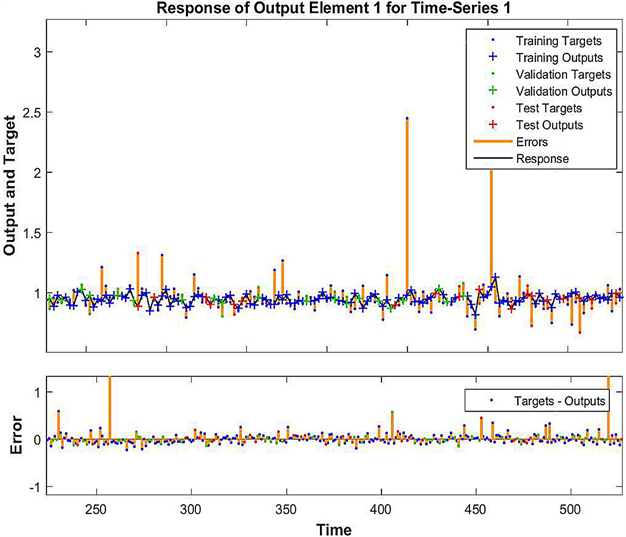Figure 3. HRB400B, C expected and actual value comparisonFigure 4. HRB400D, C expected and actual value comparison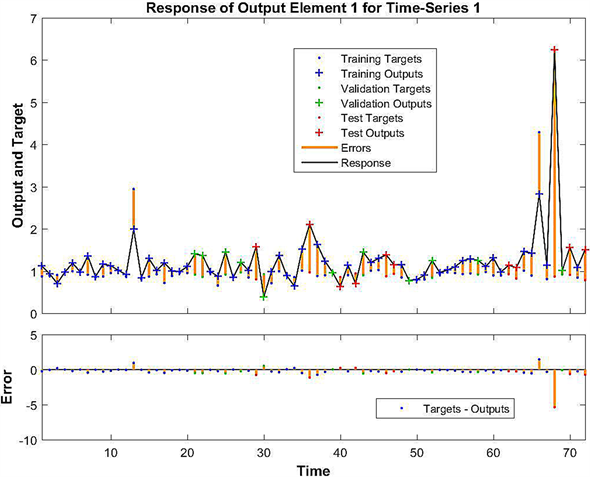Figure 5. Comparison of expected and actual values of HEB500 and C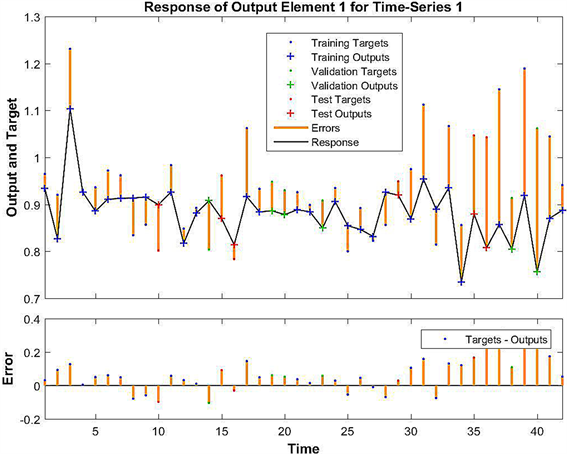Figure 6. Comparison of expected and actual values of 20MnK and C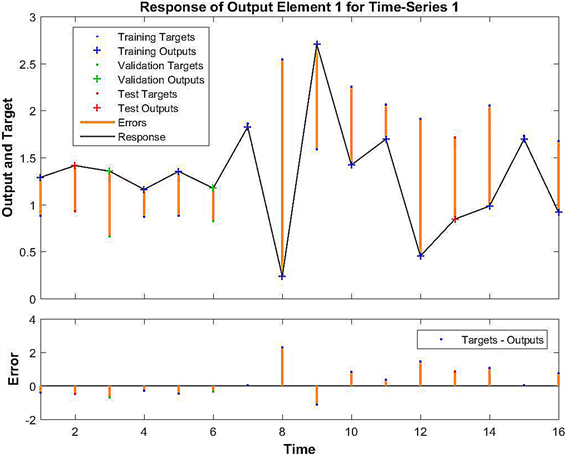Figure 7. Q, C comparison of expected and actual valuesTable 15. Part C forecasted yieldTable 16. Mn partial predicted yield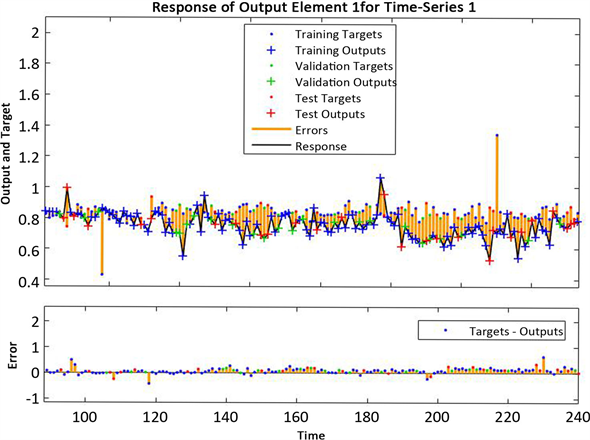Figure 8. HRB400B, Mn expected and actual value comparison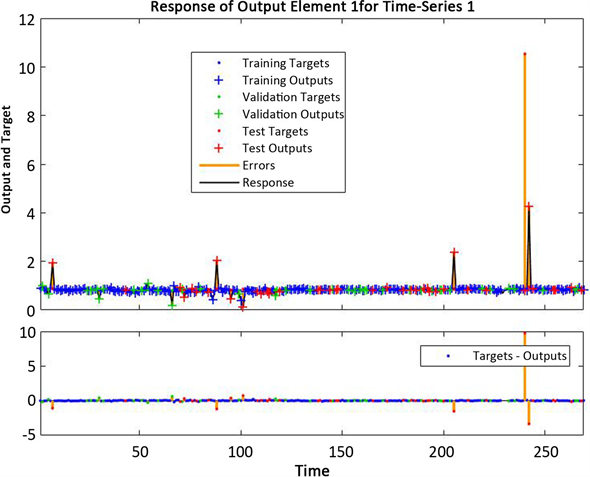Figure 9. HRB400D, Mn expectedand actual value comparison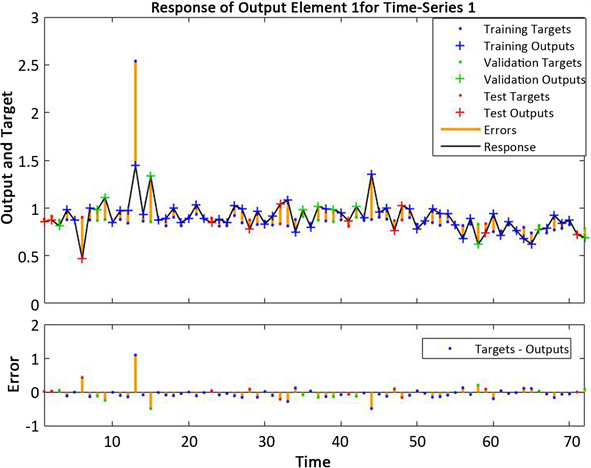Figure 10. HEB500, Mn expected value & actual value comparison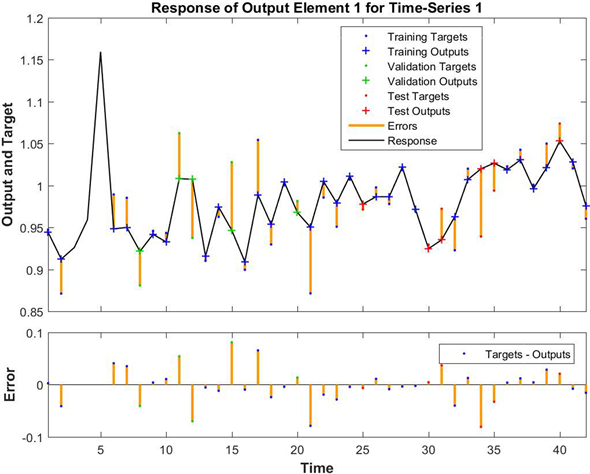Figure 11. 20MnK, Mn expected value & actual value comparison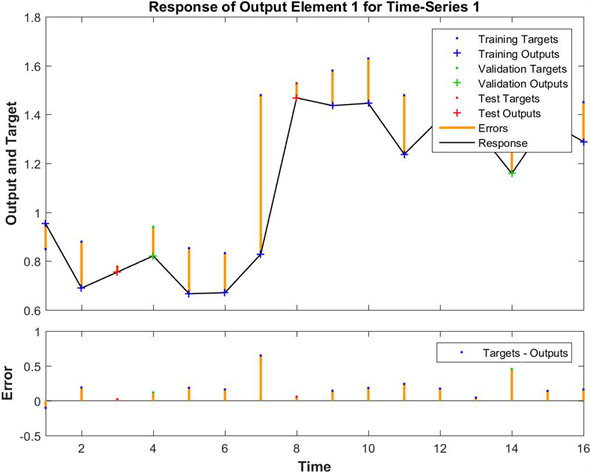Figure 12. Q, Mn expected value and actual value comparison

4.4. 分数阶灰色神经网络组合预测模型Table 17. Part C forecasted yieldTable 18. Mn partial predicted yield

4.5. 反解收得率计算模型和动态规划数学模型求解结果

4.5.1. C元素下合金配料及成本

Table 19. Alloying scheme and cost under C element

4.5.2. Mn元素下合金配料及成本Table 20. Alloying scheme and cost under Mn element

5. 总结

1) 本文利用主成分分析法对影响C、Mn两种元素的历史收得率的主要因素进行分析，通过模型的建立选出少量综合指标，巧妙地反映高效有利的历史数据信息。将历史数据进行预处理，删除失真数据，利用均值填充遗失数据，最后根据钢号的不同将其分为五大类，极大的提高了算法的高效性。

2) 针对于BP神经网络模型运算速度缓慢和易陷入局部最小值的缺点，所以本文运用分数阶灰色模型与BP神经网络网络组合的方式对预测模型进行了改进，建立了分数阶灰色神经网络组合预测模型。进一步提升了预测的精度。

3) 本文通过模型的建立对历史数据的分析，得到较为理想的合金收得率的预测结果，在一定的程度上将会提高炼钢厂的钢铁生产量及其产品质量，同时为其节省成本。将其进行推广，将有利于我国钢铁事业的发展。

 李树江, 高宪文, 柴天佑. 神经网络在钢包精炼炉配料中应用[C]//中国自动化学会. 中国控制会议论文集: 1997年卷. 庐山: 中国控制会议, 1997: 1127-1130.

 聂雨青. 20MnSi钢脱氧合金化工艺优化的研究[J]. 金属材料与冶金工程, 2000(4): 18-21.

 龚伟, 姜周华, 郑万. 转炉冶炼过程中合金成分控制模型[J]. 东北大学学报, 2002(12): 35-37.

 张广军, 李广田, 高艳宏. 特殊钢冶炼配料及合金化计算模型[J]. 材料与冶金学报, 2004(2): 16-19.

 张俊伟. 钢包炉合金成分优化模型的开发[C]//中国仪器仪表学会. 中国仪器仪表学会青年学术会议: 2007年卷. 合肥: 仪器仪表学报编辑部, 2007: 1-4.

 李香平, 张红余, 李刚利. 08Al深冲钢脱氧合金工艺优化研究[J]. 金属世界, 2008(3): 20-22.

 舒遗一, 李斌, 廖明. 180t转炉出钢脱氧合金化工艺技术研究[C]//中国金属学会. 2012年全国炼钢—连铸生产技术会论文集(上). 重庆: 中国金属学会, 2012.

 徐喆. 钢包精炼炉合金添加量的优化设定与应用[D]: [博士学位论文]. 沈阳: 东北大学, 2012.

 宿永波, 王彦祥. 转炉冶炼低碳钢脱氧合金化工艺优化[C]// 中国金属学会. 2014年全国炼钢—连铸生产技术会.唐山: 中国金属学会, 2014: 130-133.

 高鹏程, 黄德胜. 提高钢水成分精度技术优化[J]. 四川冶金, 2015(3): 14-17+23.

 刘涛, 刘怡生. 宣钢精炼炉优化合金成分配料模型的应用[J]. 河北冶金, 2018(4): 46-49.

 2019年第九届MathorCup高校数学建模挑战赛—大学生竞赛—赛氪. http://www.saikr.com/mathorcup/2019

Top